Together we stand... dividers we like.

1. Automatically, based on the existence of a certain element
2. Manually, with locations decided within SignAgent

Quick note... both of these templates are based off of the "Simple Wall Directional" template, which can be found here: Create Simple Wall Directional Template (Repeating Messages).
If anything here doesn't make sense, make sure you check out that article first!

### 1. Automatic Dividers

In this particular example, we'll show you how to have dividers show up automatically based on the existence of a certain element. For this example, it will automatically show up above every arrow in the sign artwork.

### 1. Illustrator Template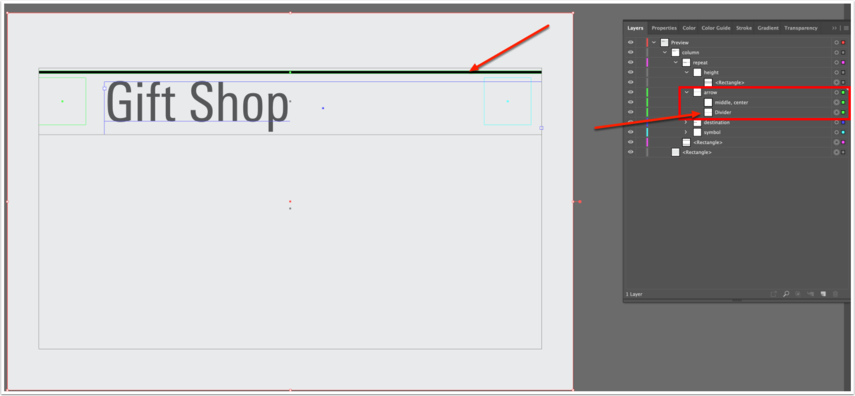To add a divider based on another element, all you need to do is add that divider within the existing sub-layer.

For example, what we did here is we added a rectangle with the dimensions and colour that we wanted, and placed its location relative to the arrow within the "height" rectangle. This rectangle is also placed within the "arrow" layer.

Now, this divider will show up in that location, relative to the height rectangle, but only when the arrow exists.

### 2. Export as SVG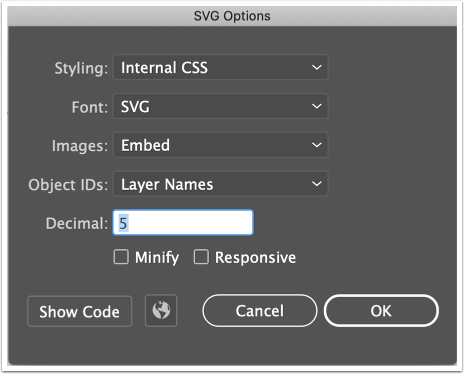Use the above settings, as normal.

### 2. Manual Dividers

In this example, we'll show you how to set up a divider field that shows up whenever you tell it to.

### 1. Illustrator Template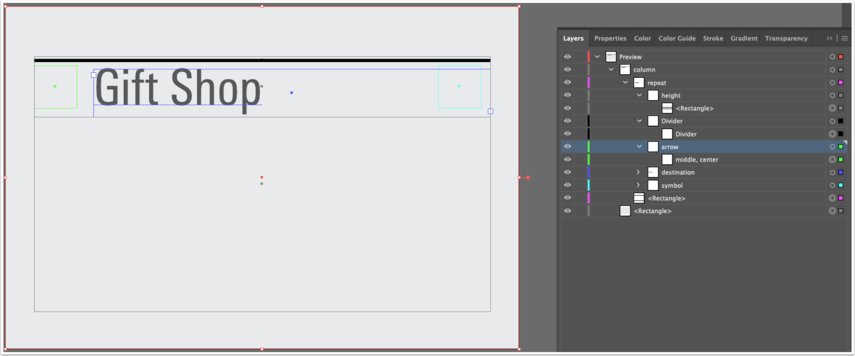Note that the main difference here is that we've added "Divider" as its own layer, with just the rectangle in it. How this will work is that, any time the Divider field has ANY content, the divider will appear. If the field is empty, the divider will not appear.

### 2. Export as SVGUse the above settings, as normal.

### 3. Set up Sign Type in SignAgent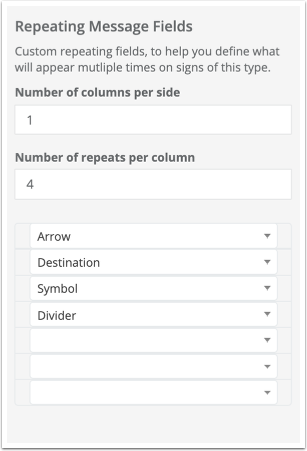Again, the main difference here is that there is now a Divider field.

Note that, in this example, I have the Divider field set up as a Text (short, with suggestions), but it could be any sort of field.

### 4. Result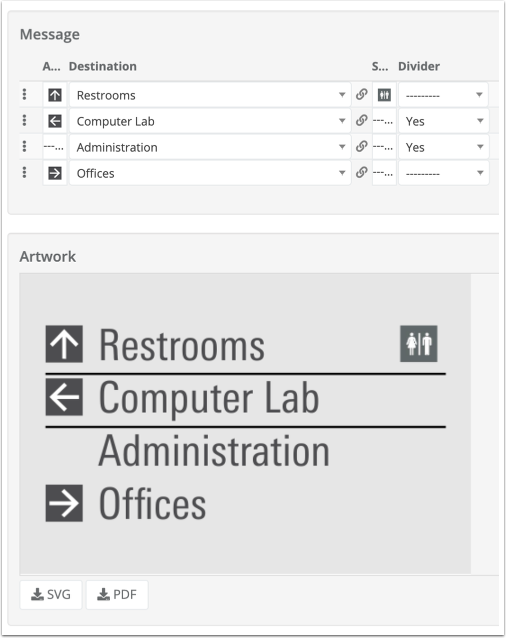Complete! Note that the divider bar is showing up here wherever it's specified in the Divider field.

Note again that I'm using "Yes" in my Divider field, but this content could be anything. As long as the field isn't empty, the divider will show up.